Want to share your content on R-bloggers? click here if you have a blog, or here if you don't.

Sixth post of our series on classification from scratch. The latest one was on the lasso regression, which was still based on a logistic regression model, assuming that the variable of interest $Y$ has a Bernoulli distribution. From now on, we will discuss technique that did not originate from those probabilistic models, even if they might still have a probabilistic interpretation. Somehow. Today, we will start with neural nets.

Maybe I should start with a disclaimer. The goal is not to replicate well designed R functions, used for predictive modeling. It is simply to get a basic understanding of what’s going on.

## Networs, nodes and edges

First of all, neurals nets are nets, or networks. I will skip the parallel with “neural” stuff because it does not help me understanding what is happening (all apologies for my poor knowledge on biology, and cells)So, it’s about some network. Networks have nodes, and edges (possibly connected) that connect nodes,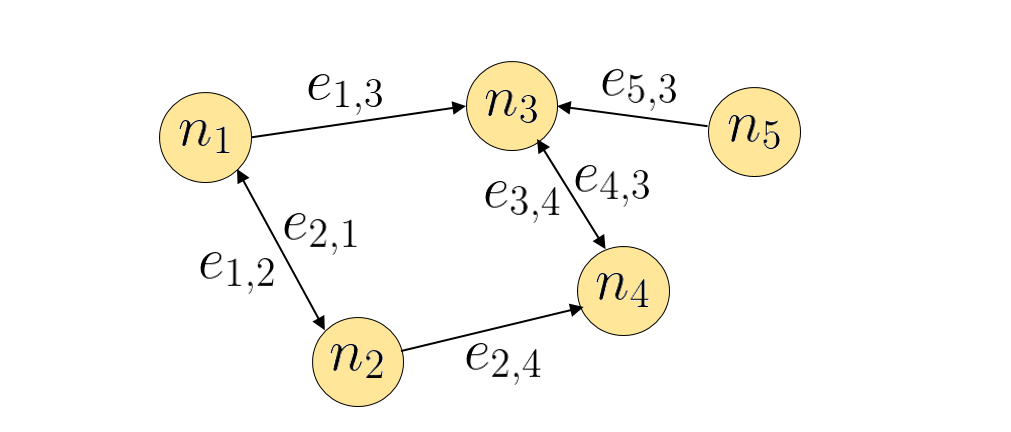or maybe, to more specific (at least it helped me understanding what’s going on), some sort of flow network,In such a network, we usually have sources (here multiple) sources (here $\color{red}\{s_1,s_2,s_3\}$), on the left, on a sink (here $\{\color{blue}t\}$), on the right. To continue with this metaphorical introduction, information from the sources should reach the sink. An usually, sources are explanatory variables, $\{\mathbf{x}_1,\cdots,\mathbf{x}_p\}$, and the sink is our variable of interest $\mathbf{y}$. And we want to create a graph, from the sources to the sink. We will have directed edges, with only one (unique) direction, where we will put weights. It is not a flow, the parallel with flow will stop here. For instance, the most simple network will be the following one, with no layer (i.e no node between the source and the sink)The output here is a binary variable $y\in\{0,1\}$ (it can also be $y\in\{-1,+1\}$ but here, it’s not a big deal). In our network, our output will be $y\in(0,1)$, because it is more easy to handly. For instance, consider $y=f($something$)$, for some function $f$ taking values in $(0,1)$. One can consider the sigmoid function[latex display=”true”]f(x)=\frac{1}{1+e^{-x}}=\frac{e^{x}}{e^{x}+1}[/latex]which is actually the logistic function (so we should not be surprised to have results somehow close the logistic regression…). This function $f$ is called the activation function, and there are thousands of such functions. If $y\in\{-1,+1\}$, people consider the hyperbolic tangent[latex display=”true”]f(x)=\tanh(x)={\frac {(e^{x}-e^{-x})}{(e^{x}+e^{-x})}}[/latex]or the inverse tangent function
[latex display=”true”]f(x)=\tan ^{-1}(x)[/latex]And as input for such function, we consider a weighted sum of incoming nodes. So here[latex display=”true”]y_i=f\left(\sum_{j=1}^p\omega_j x_{j,i}\right)[/latex]We can also add a constant actually[latex display=”true”]y_i=f\left(\omega_0+\sum_{j=1}^p\omega_j x_{j,i}\right)[/latex]So far, we are not far away from the logistic regression. Except that our starting point was a probabilistic model, in the sense that the later was interpreted as a probability (the probability that $Y=1$) and we wanted the model with the highest likelihood. But we’ll talk about selection of weights later one. First, let us construct our first (very simple) neural network. First, we have the sigmoid function

sigmoid = function(x) 1 / (1 + exp(-x))

The consider some weights. In our model with seven explanatory variables, with need 7 weights. Or 8 if we include the constant term. Let us consider $\mathbf{\omega}=\mathbf{1}$,

weights_0 = rep(1,8)
X = as.matrix(cbind(1,myocarde[,1:7]))
y_5_1 = sigmoid(X %*% weights_0)

that’s kind of stupid because all our predictions are 1, here. Let us try something else. Like $\mathbf{\omega}=\widehat{\mathbf{\beta}}^{ols}$. It is optimized, somehow, but we needed something to visualize what’s going on

weights_0 = lm(PRONO~.,data=myocarde)$coefficients then use y_5_1 = sigmoid(X %*% weights_0) In order to see if we get a “good” prediction, let use plot the ROC curve, and compare it with the one we got with a (simple) logistic regression library(ROCR) pred = ROCR::prediction(y_5_1,myocarde$PRONO)
perf = ROCR::performance(pred,"tpr", "fpr")
plot(perf,col="blue",lwd=2)
y_0 = predict(reg,type="response")
pred0 = ROCR::prediction(y_0,myocarde$PRONO) perf0 = ROCR::performance(pred0,"tpr", "fpr") plot(perf0,add=TRUE,col="red")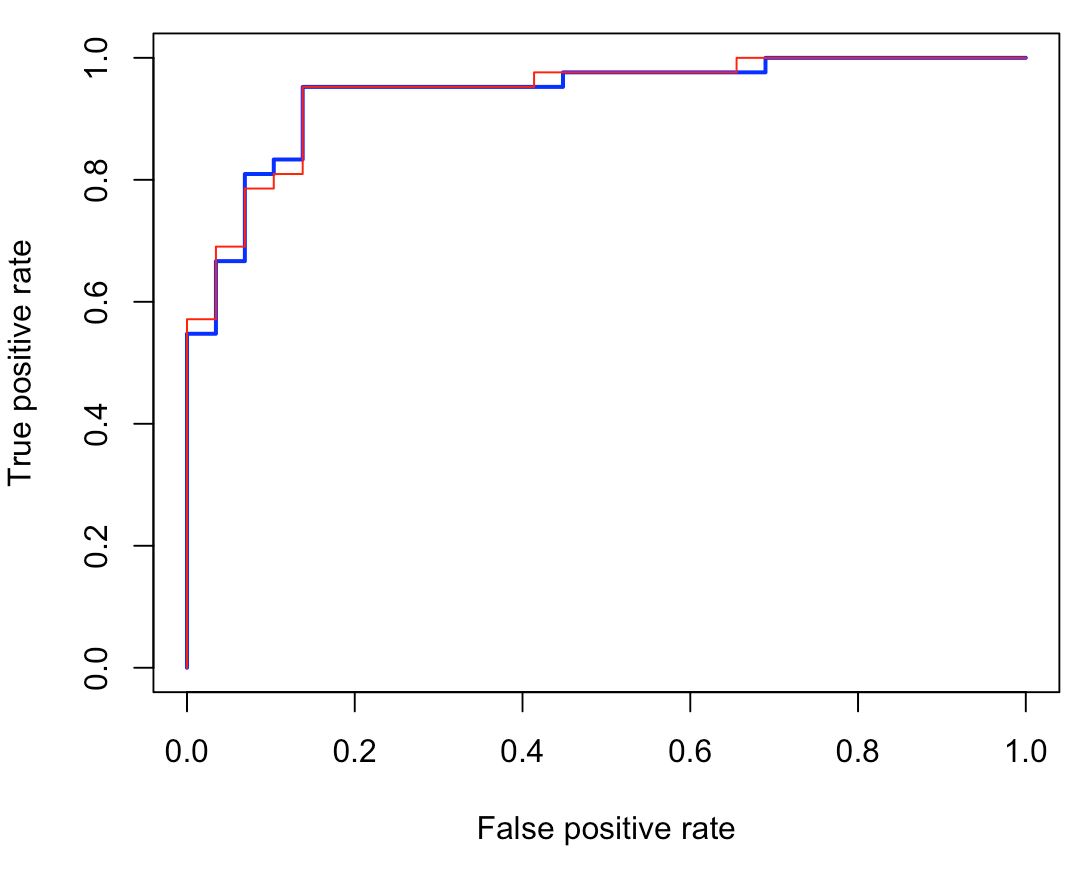That’s not bad for a very first attempt. Except that we’ve been cheating here, since we did use $\mathbf{\omega}=\widehat{\mathbf{\beta}}^{ols}$. How, for real, should we choose those weights? ## Using a loss function Well, if we want an “optimal” set of weights, we need to “optimize” an objective function. So we need to quantify the loss of a mistake, between the prediction, and the observation. Consider here a quadratic loss function loss = function(weights){ mean( (myocarde$PRONO-sigmoid(X %*% weights))^2) }

It might be stupid to use a quadratic loss function for a classification, but here, it’s not the point. We just want to understand what is the algorithm we use, and the loss function $\ell$ is just one parameter. Then we want to solve[latex display=”true”]\mathbf{\omega}^\star=\text{argmin}\left\lbrace\frac{1}{n}\sum_{i=1}^n\ell\left(y_i,f(\omega_0+\mathbf{x}_i^T\mathbf{\omega})\right)\right\rbrace[/latex]Thus, consider

weights_1 = optim(weights_0,loss)$par (where the starting point is the OLS estimate). Again, to see what’s going on, let us visualize the ROC curve y_5_2 = sigmoid(X %*% weights_1) pred = ROCR::prediction(y_5_2,myocarde$PRONO)
perf = ROCR::performance(pred,"tpr", "fpr")
plot(perf,col="blue",lwd=2)
plot(perf0,add=TRUE,col="red")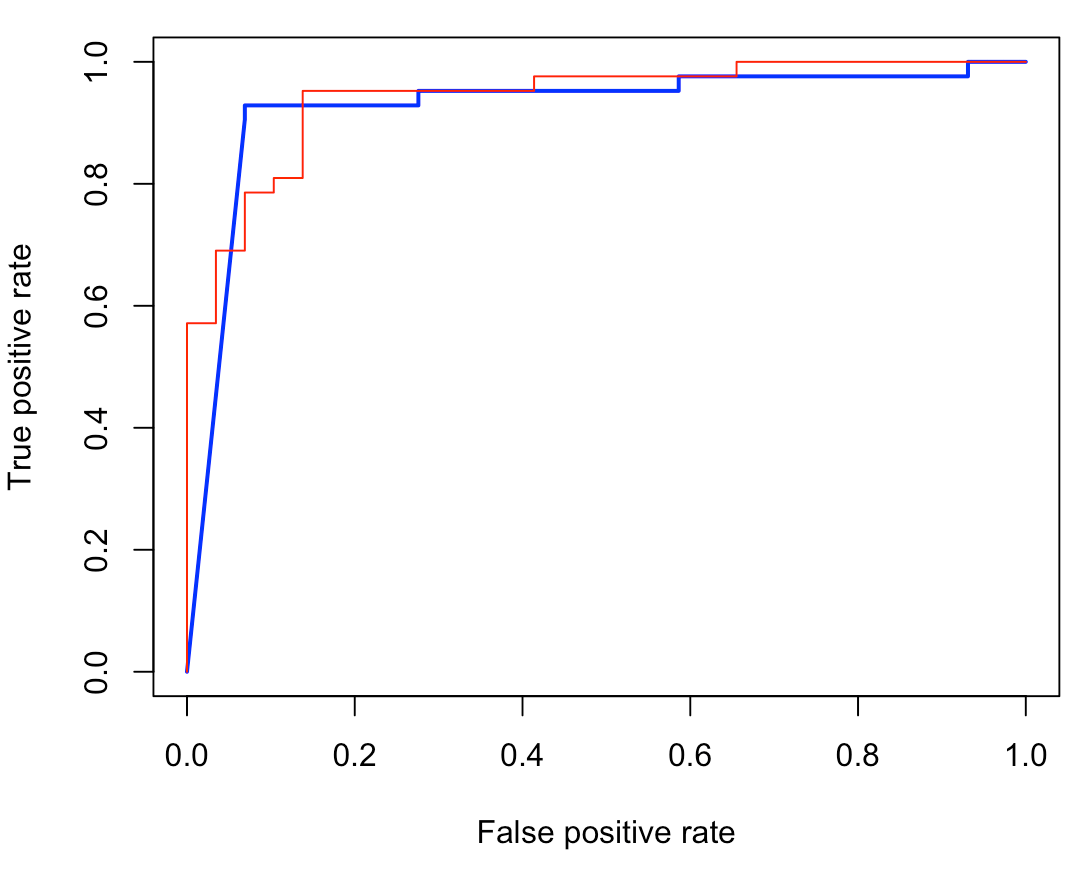That’s not amazing, but again, that’s only a first step.

## A single layer

Let us add a single layer in our network.Those nodes are connected to the sources (incoming from sources) from the left, and then connected to the sink, on the right. Those nodes are not inter-connected. And again, for that network, we need edges (i.e series of weights). For instance, on the network above, we did add one single layer, with (only) three nodes.

For such a network, the prediction formula is [latex display=”true”]\mathbf{y}=f\left( \omega_0+ \sum_{h=1}^3\omega_h f_h\left(\omega_{h,0}+ \sum_{j=1}^p \omega_{h,j} x_j\right)\right)[/latex]or more synthetically[latex display=”true”]\mathbf{y}=f\left( \omega_0+ \sum_{h=1}^3 \omega_hf_h\left(\omega_{h,0}+ \mathbf{x}^T\mathbf{\omega}_h\right)\right)[/latex]Usually, we consider the same activation function everywhere. Don’t ask me why, I find that weird.

Now, we have a lot of weights to choose. Let us use again OLS estimates

weights_1 <- lm(PRONO~1+FRCAR+INCAR+INSYS+PAPUL+PVENT,data=myocarde)$coefficients X1 = as.matrix(cbind(1,myocarde[,c("FRCAR","INCAR","INSYS","PAPUL","PVENT")])) weights_2 <- lm(PRONO~1+INSYS+PRDIA,data=myocarde)$coefficients
X2=as.matrix(cbind(1,myocarde[,c("INSYS","PRDIA")]))
weights_3 <- lm(PRONO~1+PAPUL+PVENT+REPUL,data=myocarde)$coefficients X3=as.matrix(cbind(1,myocarde[,c("PAPUL","PVENT","REPUL")])) In that case, we did specify edges, and which sources (explanatory variables) should be used for each additional node. Actually, here, other techniques could be have been used, like using a PCA. Each node will then be one of the components. But we’ll use that idea later one… X = cbind(sigmoid(X1 %*% weights_1), sigmoid(X2 %*% weights_2), sigmoid(X3 %*% weights_3)) But we’re not done here. Those were weights from the source to the know nodes, in the layer. We still need the weights from the nodes to the sink. Here, let use use a simple average weights = c(1/3,1/3,1/3) y_5_3 <- sigmoid(X %*% weights) Again, we can plot the ROC curve to see what we’ve done… pred = ROCR::prediction(y_5_3,myocarde$PRONO)
perf = ROCR::performance(pred,"tpr", "fpr")
plot(perf,col="blue",lwd=2)
plot(perf0,add=TRUE,col="red")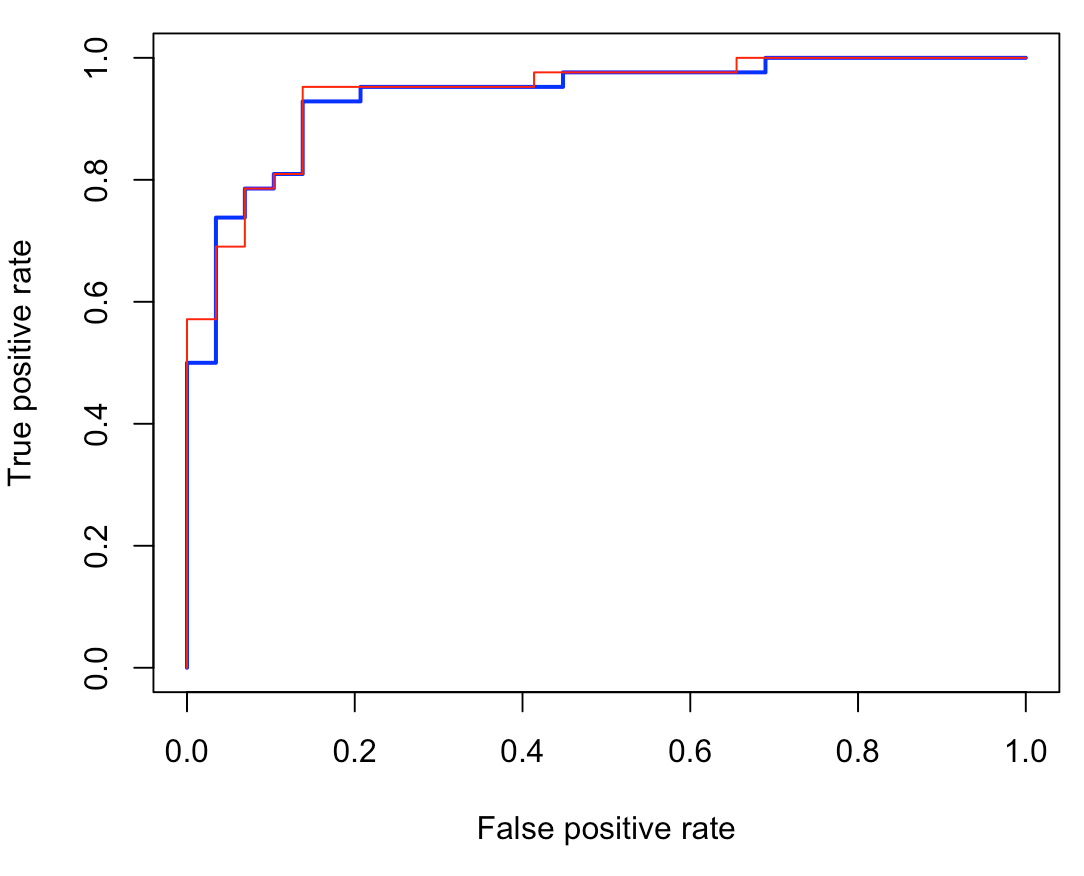## On back propagation

Now, we need some optimal selection of those weights. Observe that with only 3 nodes, there are already $(7+1)\times3+3=27$ parameters in that model! Clearly, parcimony is not the major issue when you start using neural nets! If [latex display="true"]p(\mathbf{x})=f\left( \omega_0+ \sum_{h=1}^3 \omega_hf_h\left(\omega_{h,0}+ \mathbf{x}^T\mathbf{\omega}_h\right)\right)[/latex]we want to solve[latex display="true"]\mathbf{\omega}^\star=\text{argmin}\left\lbrace\frac{1}{n}\sum_{i=1}^n\ell\left(y_i,p(\mathbf{x}_i)\right)\right\rbrace[/latex]for some loss function, which is[latex display="true"]\mathbf{\omega}^\star=\text{argmin}\left\lbrace\frac{1}{n}\sum_{i=1}^n (y_i-p(\mathbf{x}_i))^2 \right\rbrace[/latex]for the quadratic norm, or[latex display="true"]\mathbf{\omega}^\star=\text{argmin}\left\lbrace\frac{1}{n}\sum_{i=1}^n (y_i\log p(\mathbf{x}_i)+[1-y_i]\log [1-p(\mathbf{x}_i)]) \right\rbrace[/latex]if we want to use cross-entropy.

For convenience, let us center all the variable we create, otherwise, we get numerical problems.

center = function(z) (z-mean(z))/sd(z)
loss = function(weights){
weights_1 = weights[0+(1:7)]
weights_2 = weights[7+(1:7)]
weights_3 = weights[14+(1:7)]
weights_  = weights[21+1:4]
X1=X2=X3=as.matrix(myocarde[,1:7])
Z1 = center(X1 %*% weights_1)
Z2 = center(X2 %*% weights_2)
Z3 = center(X3 %*% weights_3)
X = cbind(1,sigmoid(Z1), sigmoid(Z2), sigmoid(Z3))
mean( (myocarde$PRONO-sigmoid(X %*% weights_))^2)} Now that we have our objective function, consider some starting points. We can consider weights from a PCA, and then use a gradient descent algorithm, pca = princomp(myocarde[,1:7]) W = get_pca_var(pca)$contrib
weights_0 = c(W[,1],W[,2],W[,3],c(-1,rep(1,3)/3))
weights_opt = optim(weights_0,loss)$par The prediction is then obtained using weights_1 = weights_opt[0+(1:7)] weights_2 = weights_opt[7+(1:7)] weights_3 = weights_opt[14+(1:7)] weights_ = weights_opt[21+1:4] X1=X2=X3=as.matrix(myocarde[,1:7]) Z1 = center(X1 %*% weights_1) Z2 = center(X2 %*% weights_2) Z3 = center(X3 %*% weights_3) X = cbind(1,sigmoid(Z1), sigmoid(Z2), sigmoid(Z3)) y_5_4 = sigmoid(X %*% weights_) And as previously, why not plot the ROC curve of that model pred = ROCR::prediction(y_5_4,myocarde$PRONO)
perf = ROCR::performance(pred,"tpr", "fpr")
plot(perf,col="blue",lwd=2)
plot(perf,add=TRUE,col="red")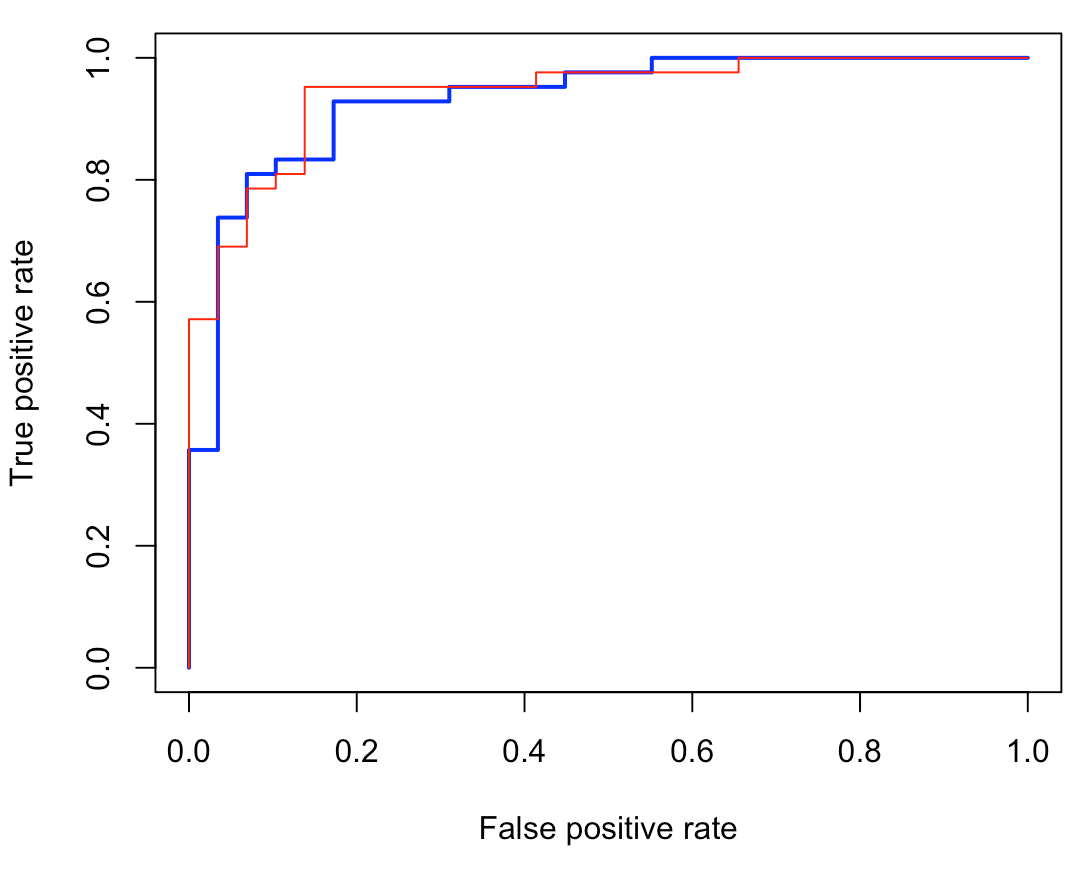That’s not too bad. But with 27 coefficients, that’s what we would expect, no?

## Using nnet() function

That’s more or less what is done in neural nets functions. Let us now have a look at some dedicated R functions.

library(nnet)
myocarde_minmax = myocarde
minmax = function(z) (z-min(z))/(max(z)-min(z))
for(j in 1:7) myocarde_minmax[,j] = minmax(myocarde_minmax[,j])

Here, variables are linearly transformed, to take values in $(0,1)$. Then we can construct a neural network with one single layer, and three nodes,

model_nnet = nnet(PRONO~.,data=myocarde_minmax,size=3)
summary(model_nnet)
a 7-3-1 network with 28 weights
options were -
b->h1 i1->h1 i2->h1 i3->h1 i4->h1 i5->h1 i6->h1 i7->h1
-9.60  -1.79  21.00  14.72 -20.45  -5.05  14.37 -17.37
b->h2 i1->h2 i2->h2 i3->h2 i4->h2 i5->h2 i6->h2 i7->h2
4.72   2.83  -3.37  -1.64   1.49   2.12   2.31   4.00
b->h3 i1->h3 i2->h3 i3->h3 i4->h3 i5->h3 i6->h3 i7->h3
-0.58  -6.03  25.14  18.03  -1.19   7.52 -19.47 -12.95
b->o  h1->o  h2->o  h3->o
-1.32  29.00 -10.32  26.27

Here, it is the complete full network. And actually, there are (online) some functions that can he used to visualize that network

library(devtools)
source_url('https://gist.githubusercontent.com/fawda123/7471137/raw/466c1474d0a505ff044412703516c34f1a4684a5/nnet_plot_update.r')
plot.nnet(model_nnet)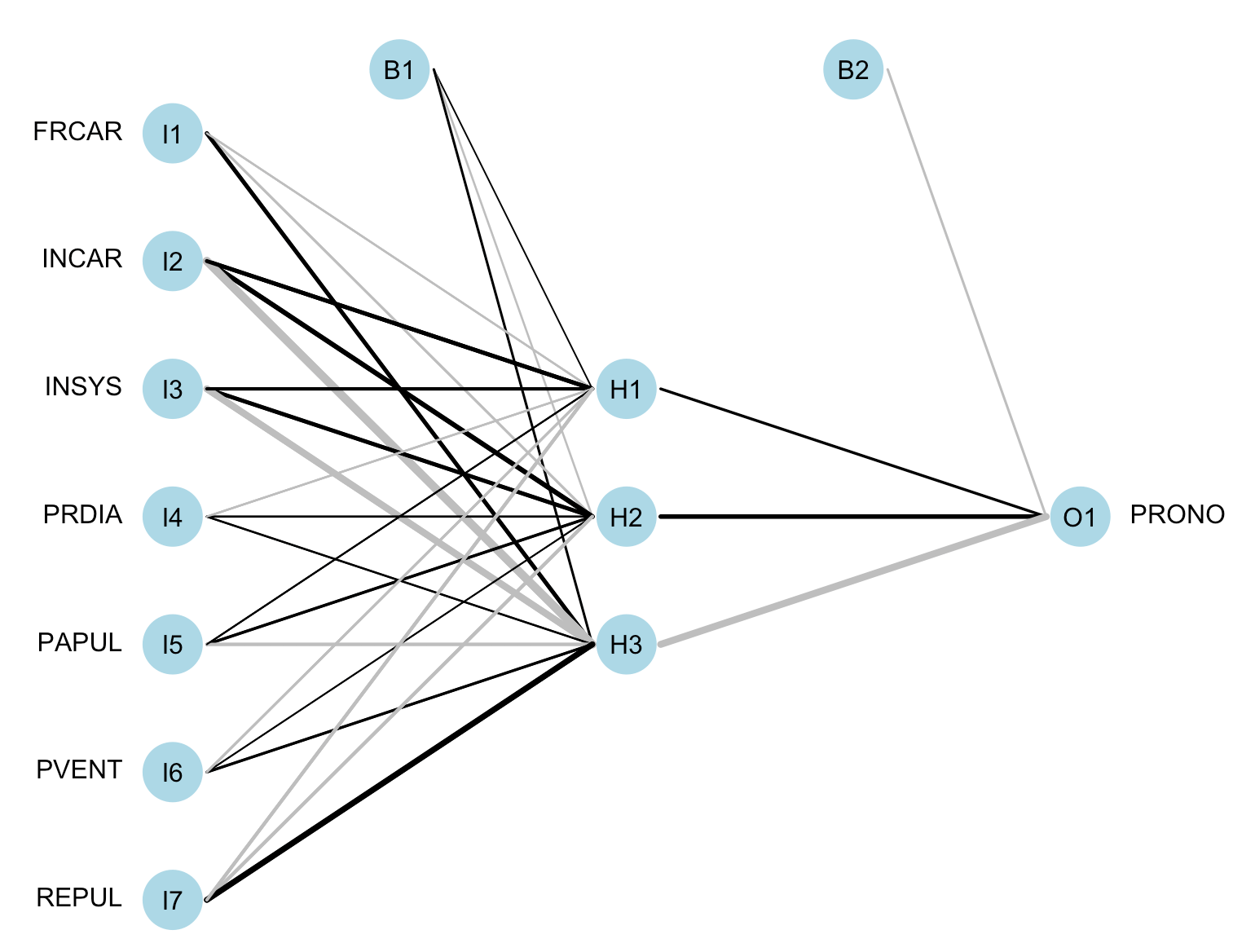Nice, isn’t it? We clearly see the intermediary layer, with three nodes, and on top the constants. Edges are the plain lines, the darker, the heavier (in terms of weights).

## Using neuralnet()

Other R functions can actually be considered.

library(neuralnet)
model_nnet = neuralnet(formula(glm(PRONO~.,data=myocarde_minmax)),
myocarde_minmax,hidden=3, act.fct = sigmoid)
plot(model_nnet)Again, for the same network structure, with one (hidden) layer, and three nodes in it.

## Network with multiple layers

The good thing is that it’s not possible to add more layers. Like two layers. Nodes from the first layer are no longuer connected with the sink, but with nodes in the second layer. And those nodes will then be connected to the sink. We now have something like
[latex display="true"]p(\mathbf{x})=f\left( \omega_0+ \sum_{h=1}^3 \omega_h f_h\left(\omega_{h,0}+ \mathbf{z}_h^T\mathbf{\omega}_h\right)\right)[/latex]where[latex display="true"]\mathbf{z}_h=f\left( \omega_{h,0}+ \sum_{j=1}^{k_h} \omega_{h,j} f_{h,j}\left(\omega_{h,j,0}+ \mathbf{x}^T\mathbf{\omega}_{h,j}\right)\right)[/latex]I may be rambling here (a little bit) but that’s a lot of parameters. Here is the visualization of such a network,

library(neuralnet)
model_nnet = neuralnet(formula(glm(PRONO~.,data=myocarde_minmax)),
myocarde_minmax,hidden=3, act.fct = sigmoid)
plot(model_nnet)## Application

Let us get back on our simple dataset, with only two covariates.

library(neuralnet)
df_minmax =df
df_minmax$y=(df_minmax$y=="1")*1
minmax = function(z) (z-min(z))/(max(z)-min(z))
for(j in 1:2) df_minmax[,j] = minmax(df[,j])
X = as.matrix(cbind(1,df_minmax[,1:2]))

Consider only one layer, with two nodes

model_nnet = neuralnet(formula(lm(y~.,data=df_minmax)),
df_minmax,hidden=c(2))
plot(model_nnet)Here, we did not specify it, but the activation function is the sigmoid (actually, it is called logistic here)

model_nnet$act.fct function (x) { 1/(1 + exp(-x)) } attr(,"type")  "logistic" f=model_nnet$act.fct

The weights (on the figure) can be obtained using

w0 = model_nnet$weights[][][,1] w1 = model_nnet$weights[][][,1]
w2 = model_nnet$weights[][][,2] Now, to get our prediction, we should use[latex display="true"]p(\mathbf{x})=f\left( \omega_0+ \omega_1 f(\omega_{1,0}+ \mathbf{x}_h^T\mathbf{\omega}_{1,1:2})+\omega_1 f(\omega_{2,0}+ \mathbf{x}_h^T\mathbf{\omega}_{2,1:2})\right)[/latex]which can be obtained using f(cbind(1,f(X%*%w1),f(X%*%w2))%*%w0) [,1] [1,] 0.7336477343 [2,] 0.7317999050 [3,] 0.7185803540 [4,] 0.7404005280 [5,] 0.7518482779 [6,] 0.4939774149 [7,] 0.4965876378 [8,] 0.7101714888 [9,] 0.5050760026 [10,] 0.5049877644 Unfortunately, it is not the output of the model here, neuralnet::prediction(model_nnet) Data Error: 0;$rep1
x1           x2              y
1  0.1250 0.0000000000  0.02030470787
2  0.0625 0.1176470588  0.89621706711
3  0.9375 0.2352941176  0.01995171956
4  0.0000 0.4705882353  1.10849420363
5  0.5000 0.4705882353 -0.01364966058
6  0.3125 0.5294117647 -0.02409150561
7  0.6875 0.8235294118  0.93743057765
8  0.3750 0.8823529412  1.01320924782
9  1.0000 0.9058823529  1.04805134309
10 0.5625 1.0000000000  1.00377379767

If anyone has a clue, I’d be glad to know what went wrong here… I find that odd to have outputs outside the $(0,1)$ interval, but the output is neither[latex display="true"]p(\mathbf{x})=\omega_{0,0}+ \omega_{0,1} f(\omega_{1,0}+ \mathbf{x}_h^T\mathbf{\omega}_{1,1:2})+\omega_{0,2} f(\omega_{2,0}+ \mathbf{x}_h^T\mathbf{\omega}_{2,1:2})[/latex]

cbind(1,f(X%*%w1),f(X%*%w2))%*%w0
[,1]
[1,]  1.01320924782
[2,]  1.00377379767
[3,]  0.93743057765
[4,]  1.04805134309
[5,]  1.10849420363
[6,] -0.02409150561
[7,] -0.01364966058
[8,]  0.89621706711
[9,]  0.02030470787
[10,]  0.01995171956